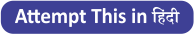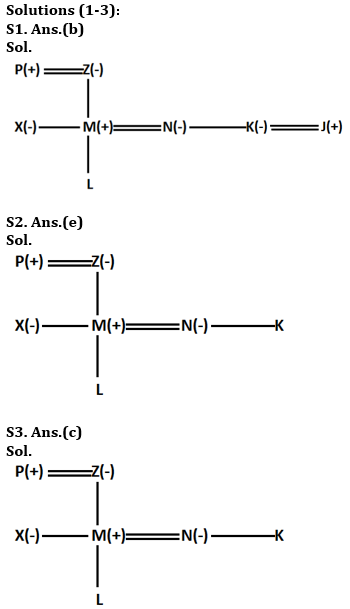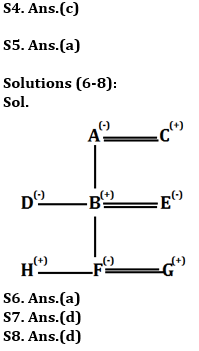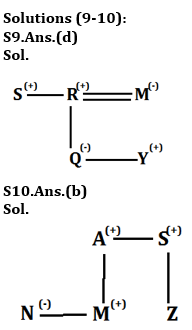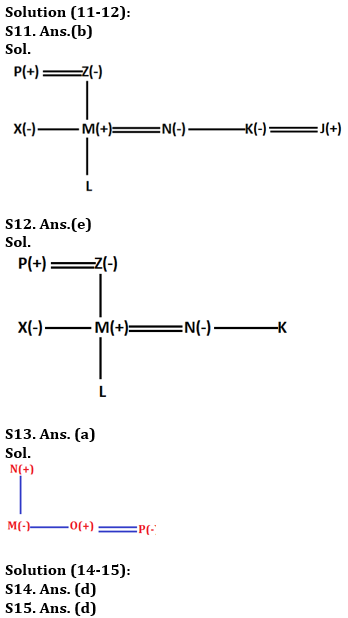Latest Banking jobs   »   Reasoning Ability, Blood Relation Quiz For...

# Reasoning Ability, Blood Relation Quiz For SBI PO, Clerk Prelims 2021- 18th June

Directions (1-3): Study the information carefully and answer the questions given below.
P is father of M who is brother in law of K. N is daughter in law of Z who is grandmother of L. P has only two children one son and one daughter. X is sister in law of N. P is father in law of N. K is unmarried.

Q1. If K is wife of J, then what could be the relation of J with respect to N?
(a) Brother
(b) Brother in law
(c) Uncle
(d) Cannot be determined
(e) None of these

Q2. What is relation of L with respect to X?
(a) Nephew
(b) Niece
(c) Son
(d) Daughter
(e) Cannot be determined

Q3. How is P related to L?
(a) Father
(b) Mother
(c) Grand father
(d) Cannot be determined
(e) None of these.

Q4. A man says to a lady, “Your father is the father in law of my father’s only child.” How is the lady related to the man?
(a) Sister-in-law
(b) Granddaughter
(c) Wife
(d) Daughter
(e) Aunt

Q5. Showing a man on the stage, Rita said, ” He is the brother of the daughter of the wife of my husband. How is the man on stage related to Rita?
(a) Son
(b) Husband
(c) Cousin
(d) Nephew
(e) Niece

Directions (6-8): Study the following information carefully to answer the following question:
There are eight members in the family having three generations. There are only three married couple. A is mother of D. G is son in law of B. H is nephew of D. C has only one son. F is granddaughter of C. E is mother of F. D is unmarried. F is a married woman.

Q6. How C is related to D?
(a) Father
(b) Mother
(c) Son
(d) Son in law
(e) Brother

Q7. Who among the following is son in law of E?
(a) A
(b) C
(c) H
(d) G
(e) None of these

Q8. Who among the following is niece of D?
(a) C
(b) E
(c) H
(d) F
(e) G

Directions (9-10): Each of these questions is based on the following information:
(i) P % Q means P is the father of B.
(ii) P @ Q means P is the sister of B.
(iii) P \$ Q means P is the brother of B.
(iv) P * Q means P is the son of B.

Q9. In the expression S \$ R % Q @ Y * M, how is M related to S?
(a) Aunt
(b) Mother
(c) Sister
(d) Sister in law
(e) None of these

Q10. In the expression N @ M * A \$ S % Z, how is S related to N?
(a) Aunt
(b) Uncle
(c) Sister
(d) Sister in law
(e) None of these

Directions (11-12): Study the information carefully and answer the questions given below.
P is father of M who is brother in law of K. N is daughter in law of Z who is grandmother of L. P has only two children one son and one daughter. X is sister in law of N. P is father in law of N. K is unmarried.

Q11. If K is wife of J then what could be the relation of J with respect to N?
(a) Brother
(b) Brother in law
(c) Uncle
(d) Cannot be determined
(e) None of these.

Q12. What is relation of L with respect to X?
(a) Nephew
(b) Niece
(c) Son
(d) Daughter
(e) Cannot be determined

Directions (13): Study the following information carefully to answer the given questions
P × Q means P is father of Q
P ÷ Q means P is daughter of Q
P + Q means P is sister of Q
P – Q means P is husband of Q

Q13. In M ÷ N × O – P, How O related to M?
(a)Brother
(b)Son –in-law
(c)Father
(d)Son
(e)None of these

Directions (14-15): Study the information carefully and answer the questions given below.
M, N, O, P, Q and R are six family members. Q is son of P, who is not the mother of Q. N is brother of P. R and P are a married couple. O is daughter of R, who is sister of M.

Q14. How many female members are there in the family?
(a) Two
(b) Three
(c) Four
(d) Can’t be determined
(e) None of these

Q15. How is Q related to O?
(a) Mother
(b) Father
(c) Sister
(d) Brother
(e) None of these

Practice More Questions of Reasoning for Competitive Exams:

###### SBI Clerk Study Plan 2021

Solutions